# Gene set co-regulation analysis tutorial

This vignette describes GESECA (gene set co-regulation analysis): a method to identify gene sets that have high gene correlation. We will show how GESECA can be used to find regulated pathways in multi-conditional data, where there is no obvious contrast that can be used to rank genes for GSEA analysis. As examples we will consider a time course microarray experiment and a spatial transcriptomics dataset.

## Overiew of GESECA method

GESECA takes as an input:

• E - gene expression matrix, where rows and columns correspond to genes and samples respectively.
• P - list of gene sets (i.e.  hallmark gene sets).

Note: genes identifier type should be the same for both elements of P and for row names of matrix E.

By default, GESECA method performs centering for rows of the matrix E. So, after that, the gene values are assumed to have zero mean. Then for each gene set p in P let us introduce the gene set score in the following form:

score <- sum(colSums(E[p, ])**2) / length(p)

This score was inspired by the variance of principal components from the principal component analysis (PCA). Therefore, the given score can be viewed in terms of explained variance by the gene set p. Geometrically, this can be considered as an embedding of samples into a one-dimensional space, given by a unit vector in which nonzero positions correspond to genes from gene set p.

In the case of row-centered matrix E the variance of highly correlated genes is summed up to a higher score. While the genes that are not correlated cancel each other and the total gene set variance is low. See the toy example: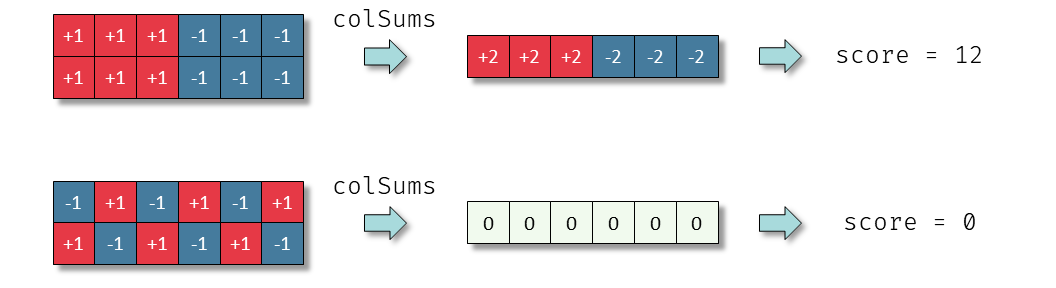Toy example of GESECA score calculation

Another major feature of the proposed score is that it does not require an explicit sample annotation or a contrast. As the result, GESECA can be applied to various types of sequencing technologies: RNA-seq, single-cell sequencing, spatial RNA-seq, etc.

To assess statistical significance for a given gene set p we calculate an empirical P-value by using gene permutations. The definition of the P-value is given by the following expression: $\mathrm{P} \left(\text{random score} \geqslant \text{score of p} \right).$ The estimation of the given P-value is done by sampling random gene sets with the same size as p from the row names of matrix E. In practice, the theoretical P-value can be extremely small, so we use the adaptive multilevel Markov Chain Monte Carlo scheme, that we used previously in fgseaMultilevel procedure. For more details, see the preprint.

## Analysis of time course data

In the first example we will consider a time course data of Th2 activation from the dataset GSE200250.

First, let prepare the dataset. We load it from Gene Expression Omnibus, apply log and quantile normalization and filter lowly expressed genes.

library(GEOquery)
library(limma)

gse200250 <- getGEO("GSE200250", AnnotGPL = TRUE)[]

es <- gse200250
es <- es[, grep("Th2_", es$title)] es$time <- as.numeric(gsub(" hours", "", es$time point:ch1)) es <- es[, order(es$time)]

exprs(es) <- normalizeBetweenArrays(log2(exprs(es)), method="quantile")

es <- es[order(rowMeans(exprs(es)), decreasing=TRUE), ]
es <- es[!duplicated(fData(es)$Gene ID), ] rownames(es) <- fData(es)$Gene ID
es <- es[!grepl("///", rownames(es)), ]
es <- es[rownames(es) != "", ]

fData(es) <- fData(es)[, c("ID", "Gene ID", "Gene symbol")]

es <- es[head(order(rowMeans(exprs(es)), decreasing=TRUE), 12000), ]
#>       GSM6025497 GSM6025506 GSM6025498 GSM6025507 GSM6025499 GSM6025508
#> 20042   15.95540   15.98219   15.98219   16.00741   15.93798   15.96460
#> 20005   15.93039   15.96460   15.90505   15.91366   15.95540   15.72169
#> 20088   15.93798   15.91054   15.84485   15.87839   15.92647   15.91366
#> 20102   15.88375   15.78037   15.82486   15.87013   15.98219   15.94702
#> 20103   15.84885   15.86085   15.77502   15.84141   15.72169   15.85271
#> 20090   15.91054   15.80253   15.93798   15.74269   15.96460   15.90505
#>       GSM6025500 GSM6025509 GSM6025501 GSM6025510 GSM6025502 GSM6025511
#> 20042   15.93039   16.00741   15.94702   16.00741   15.92647   15.98219
#> 20005   15.89942   15.94702   15.95540   15.79744   15.91366   15.80778
#> 20088   15.84485   15.92647   15.86573   15.86573   15.80778   15.88972
#> 20102   15.75168   15.90505   15.79744   15.78575   15.91054   15.89411
#> 20103   15.86085   15.82057   15.91054   15.91981   15.91981   15.70228
#> 20090   15.84885   15.88375   15.76562   15.82057   15.76024   15.82057
#>       GSM6025503 GSM6025512 GSM6025504 GSM6025513 GSM6025505 GSM6025514
#> 20042   15.98219   16.00741   15.96460   16.00741   16.00741   16.00741
#> 20005   15.90505   15.84485   15.85590   15.78575   15.83803   15.89411
#> 20088   15.82486   15.83803   15.86573   15.93039   15.85271   15.87839
#> 20102   15.86085   15.75168   15.88972   15.94702   15.91054   15.88972
#> 20103   15.84141   15.93039   15.90505   15.89942   15.90505   15.91054
#> 20090   15.93798   15.83089   15.91366   15.73523   15.91981   15.84885

Then we obtain the pathway list. Here we use Hallmarks collection from MSigDB database.

library(msigdbr)
pathwaysDF <- msigdbr("mouse", category="H")
pathways <- split(as.character(pathwaysDF$entrez_gene), pathwaysDF$gs_name)

Now we can run GESECA analysis:

library(fgsea)
set.seed(1)
gesecaRes <- geseca(pathways, exprs(es), minSize = 15, maxSize = 500)

The resulting table contain GESECA scores and the corresponding P-values:

head(gesecaRes, 10)
#>  1:               HALLMARK_E2F_TARGETS 1.5886651 3.726293e-48 1.415991e-46
#>  2:                   HALLMARK_HYPOXIA 1.1041997 3.509752e-34 6.668528e-33
#>  3:            HALLMARK_G2M_CHECKPOINT 1.0281398 1.078730e-32 1.366391e-31
#>  4:            HALLMARK_MYC_TARGETS_V1 0.5788220 6.993558e-21 6.643880e-20
#>  5:                HALLMARK_GLYCOLYSIS 0.5963190 1.773982e-20 1.348227e-19
#>  6:            HALLMARK_MYC_TARGETS_V2 0.7244975 3.370652e-20 2.134746e-19
#>  7:   HALLMARK_TNFA_SIGNALING_VIA_NFKB 0.5399796 5.436774e-19 2.951391e-18
#>  8:           HALLMARK_MITOTIC_SPINDLE 0.3461497 4.304476e-13 2.044626e-12
#>  9: HALLMARK_INTERFERON_GAMMA_RESPONSE 0.2468646 6.688237e-10 2.541530e-09
#> 10:       HALLMARK_IL2_STAT5_SIGNALING 0.2528643 6.688237e-10 2.541530e-09
#>       log2err size
#>  1: 1.8030940  181
#>  2: 1.5161076  149
#>  3: 1.4815676  175
#>  4: 1.1778933  186
#>  5: 1.1690700  153
#>  6: 1.1601796   50
#>  7: 1.1239150  156
#>  8: 0.9214260  165
#>  9: 0.8012156  157
#> 10: 0.8012156  177

We can plot gene expression profile of HALLMARK_E2F_TARGETS pathway and see that these genes are strongly activated at 24 hours time point:

plotCoregulationProfile(pathway=pathways[["HALLMARK_E2F_TARGETS"]],
E=exprs(es), titles = es$title, conditions=es$time point:ch1)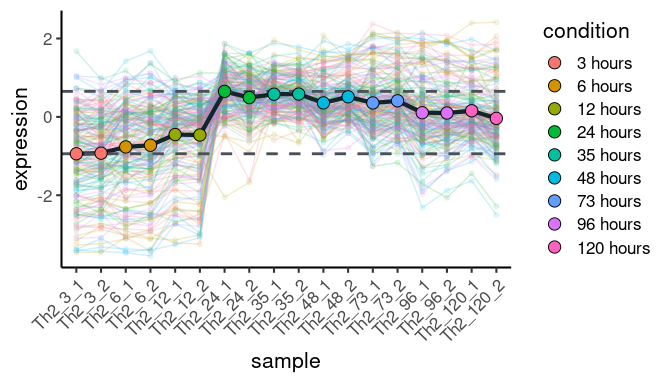Hypoxia genes have slightly different profile, getting activated around 48 hours:

plotCoregulationProfile(pathway=pathways[["HALLMARK_HYPOXIA"]],
E=exprs(es), titles = es$title, conditions=es$time point:ch1)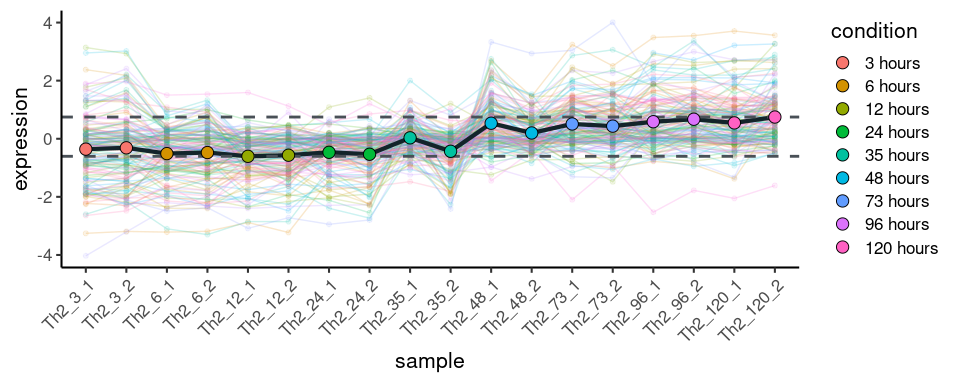To get an overview of the top pathway patterns we can use plotGesecaTable function:

plotGesecaTable(gesecaRes |> head(10), pathways, E=exprs(es), titles = es$title)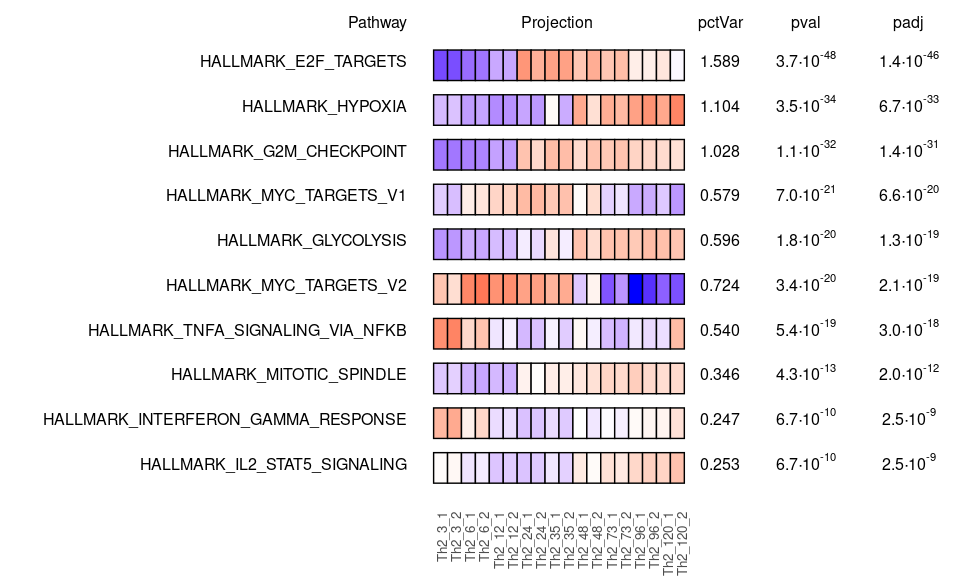When the expression matrix contains many samples, a PCA-reduced expression matrix can be used instead of the full matrix to improve the performance. Let reduce the sample space from 18 to 10 dimensions, preserving as much gene variation as possible. E <- t(base::scale(t(exprs(es)), scale=FALSE)) pcaRev <- prcomp(E, center=FALSE) Ered <- pcaRev$x[, 1:10]
dim(Ered)
#>  12000    10

Now we can run GESECA on the reduced matrix, however we need to disable automatic centering, as we already have done it before the reduction.

set.seed(1)
gesecaResRed <- geseca(pathways, Ered, minSize = 15, maxSize = 500, center=FALSE)
#>  1:               HALLMARK_E2F_TARGETS 1.6274291 1.666912e-47 6.167575e-46
#>  2:                   HALLMARK_HYPOXIA 1.1242216 4.502652e-33 5.642121e-32
#>  3:            HALLMARK_G2M_CHECKPOINT 1.0527938 4.574693e-33 5.642121e-32
#>  4:            HALLMARK_MYC_TARGETS_V2 0.7422724 8.826326e-21 6.602863e-20
#>  5:            HALLMARK_MYC_TARGETS_V1 0.5923285 8.922788e-21 6.602863e-20
#>  6:                HALLMARK_GLYCOLYSIS 0.6088536 5.051598e-20 3.115152e-19
#>  7:   HALLMARK_TNFA_SIGNALING_VIA_NFKB 0.5493606 5.551497e-18 2.934363e-17
#>  8:           HALLMARK_MITOTIC_SPINDLE 0.3530595 2.868317e-12 1.326596e-11
#>  9:       HALLMARK_IL2_STAT5_SIGNALING 0.2584534 6.767387e-10 2.782148e-09
#> 10: HALLMARK_INTERFERON_GAMMA_RESPONSE 0.2501224 1.838503e-09 6.802462e-09
#>       log2err size
#>  1: 1.7915725  181
#>  2: 1.4885397  149
#>  3: 1.4885397  175
#>  4: 1.1778933   50
#>  5: 1.1778933  186
#>  6: 1.1512205  153
#>  7: 1.0864405  156
#>  8: 0.8986712  165
#>  9: 0.8012156  177
#> 10: 0.7749390  157

The scores and P-values are similar to the ones we obtained for the full matrix.

library(ggplot2)
ggplot(data=merge(gesecaRes[, list(pathway, logPvalFull=-log10(pval))],
gesecaResRed[, list(pathway, logPvalRed=-log10(pval))])) +
geom_point(aes(x=logPvalFull, y=logPvalRed)) +
coord_fixed() + theme_classic()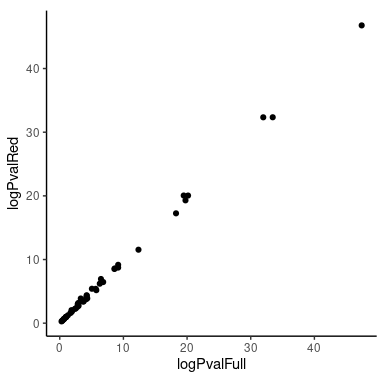## Analysis of spatial RNA-seq

As the second example we will consider a spatial transcriptomics dataset of a mouse brain.

To load the data we will use SeuratData package that can be installed with the following command:

devtools::install_github('satijalab/seurat-data')

Next we install the package with the dataset:

SeuratData::InstallData("stxBrain")

Now we can load the data:

library(Seurat)
library(SeuratData)
library(ggplot2)
library(patchwork)
brain <- LoadData("stxBrain", type = "anterior1")

We apply an appropriate normalization (note that we are using 10000 genes, which will be later used as a gene universe for the analysis):

brain <- SCTransform(brain, assay = "Spatial", verbose = FALSE, variable.features.n = 10000)

To speed up the analysis, instead of using the full transformed gene expression matrix, we will consider only its first principal components. Note that a “reverse” PCA should be done: the principal components should correspond to linear combinations of the cells, not linear combinations of the genes as in “normal” PCA. By default SCTransform returns centered gene expression, so we can run PCA directly.

brain <- RunPCA(brain, assay = "SCT", verbose = FALSE,
rev.pca = TRUE, reduction.name = "pca.rev", reduction.key="PCR_")
E <- brain@reductions$pca.rev@feature.loadings We will use KEGG pathways as the gene set collection. library(msigdbr) pathwaysDF <- msigdbr("mouse", category="C2", subcategory = "CP:KEGG") pathways <- split(pathwaysDF$gene_symbol, pathwaysDF$gs_name) Now we can run the analysis (we set center=FALSE because we use the reduced matrix): set.seed(1) gesecaRes <- geseca(pathways, E, minSize = 15, maxSize = 500, center = FALSE) Finally, let us plot spatial expression of the top four pathways:  topPathways <- gesecaRes[, pathway] |> head(4) for (ppn in topPathways) { pp <- pathways[[ppn]] pp <- intersect(pp, rownames(E)) score <- colSums(brain@assays$SCT@scale.data[pp, ])/sqrt(length(pp))
brain@meta.data[[ppn]] <- score
}
SpatialFeaturePlot(brain, features = topPathways, )